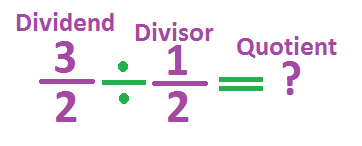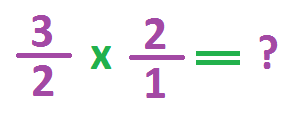# Rules for Dividing Fractions

Instructor: Allison Petrovic

Allison has experience teaching high school and college mathematics and has a master's degree in mathematics education.

In this lesson, we'll follow three simple steps to help us divide fractions. If you already know how to multiply fractions, you're on your way to learning how to divide fractions!

## Fractions in Real Life

Let's imagine that you are wrapping presents for your mother for Mother's Day. You have a long piece of ribbon that is 3/2 feet long. You need separate pieces that are 1/2 feet long. How many pieces can you cut that are 1/2 feet long from the 3/2 feet long piece of ribbon?

In order to solve this problem, we need to know how to divide fractions. We will be taking the larger number 3/2 and dividing it by the smaller fraction 1/2. Before we go over the steps on how to divide fractions, we need to go over some important definitions.

## Key Terms for Division

In any division problem, you have a dividend, divisor and quotient. Remember that we always divide the dividend by the divisor. So in our problem, 3/2 is the dividend and 1/2 is the divisor! The quotient is the answer to any division problem. In our problem, we are looking for the quotient.We need to go over one more definition before we learn the steps to dividing fractions. This may be a new word for you. Let's talk about the word reciprocal.

To get the reciprocal of any fraction, you just switch the numbers of the numerator and denominator. Think of it as turning the fraction upside down. For an example, the reciprocal of the fraction 5/8 is 8/5. Notice how we just switched the 5 and 8. Now let's go over the steps to dividing fractions.

## Step 1: Change the Operation and Take the Reciprocal

The first step to divide fractions is very important. When we divide fractions, we need to change the operation from division to multiplication. And because we are changing the operation to multiplication, we must also take the reciprocal of the divisor.

Remember that our problem is 3/2 divided by 1/2. So our divisor is 1/2. The reciprocal of 1/2 is 2/1. Remember we just switch the 1 and 2.

After changing the operation to multiplication and taking the reciprocal of the divisor, we now have 3/2 x 2/1.To unlock this lesson you must be a Study.com Member.

### Register to view this lesson

Are you a student or a teacher?

#### See for yourself why 30 million people use Study.com

##### Become a Study.com member and start learning now.
Back
What teachers are saying about Study.com

### Earning College Credit

Did you know… We have over 200 college courses that prepare you to earn credit by exam that is accepted by over 1,500 colleges and universities. You can test out of the first two years of college and save thousands off your degree. Anyone can earn credit-by-exam regardless of age or education level.